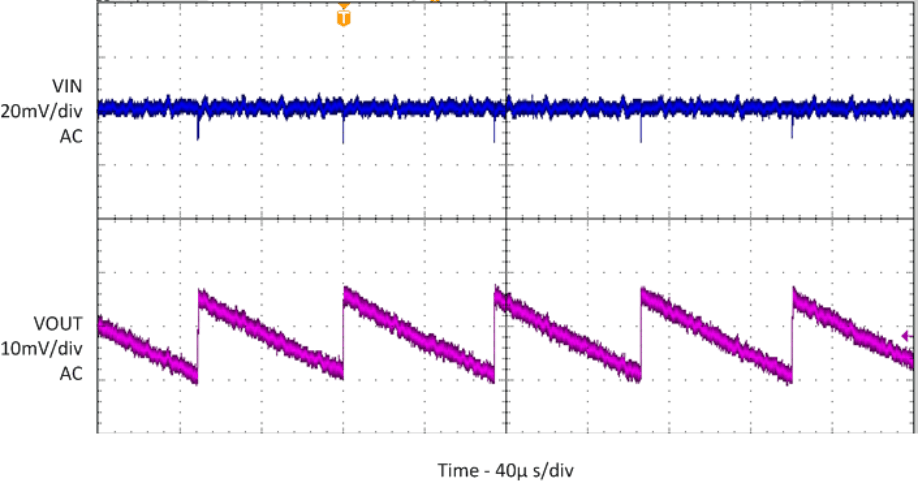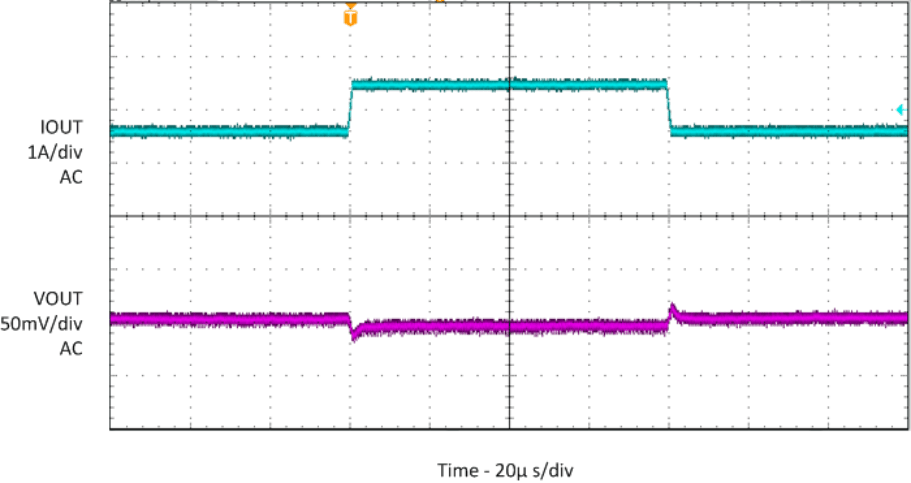SLVSDN4 June   2017

PRODUCTION DATA.

1. Features
2. Applications
3. Description
1.     Device Images
4. Revision History
5. Pin Configuration and Functions
6. Specifications
7. Detailed Description
1. 7.1 Overview
2. 7.2 Functional Block Diagram
3. 7.3 Feature Description
4. 7.4 Device Functional Modes
8. Application and Implementation
1. 8.1 Application Information
2. 8.2 Typical Applications
1. 8.2.1 1.8-V Output Application
3. 8.3 System Examples
9. Power Supply Recommendations
10. 10Layout
11. 11Device and Documentation Support
12. 12Mechanical, Packaging, and Orderable Information
1. 12.1 Package Materials Information

• SIL|8

#### 8.2.1.3 Application Performance Curves

TA = 25°C, VIN = 12 V, VOUT = 1.8 V, unless otherwise noted.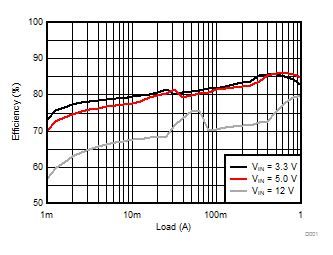Figure 6. Efficiency, VOUT = 1.0 V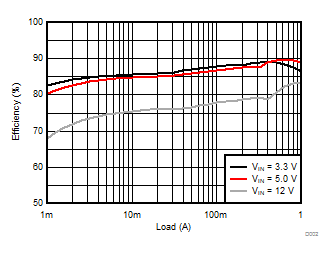Figure 8. Efficiency, VOUT = 1.8 V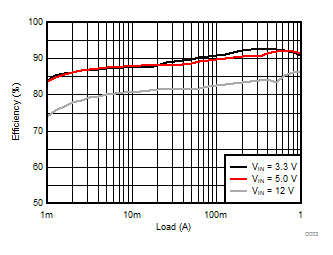Figure 10. Efficiency, VOUT = 2.5 V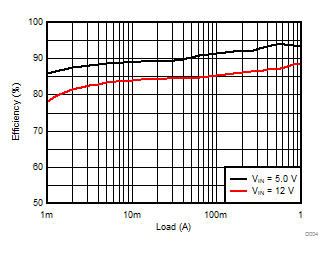Figure 12. Efficiency, VOUT = 3.3 V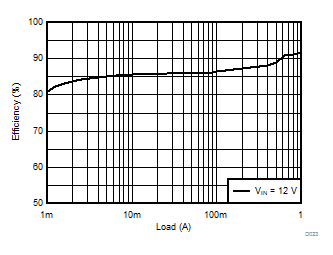Figure 14. Efficiency, VOUT = 5.0 V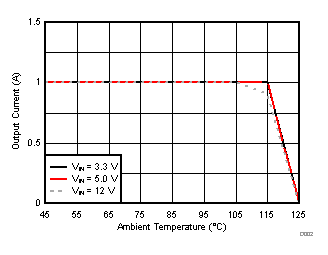θJA = 46.1 °C/W
Figure 16. Thermal Derating, VOUT = 1 V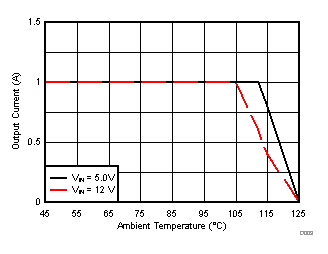θJA = 46.1 °C/W
Figure 18. Thermal Derating, VOUT = 3.3 V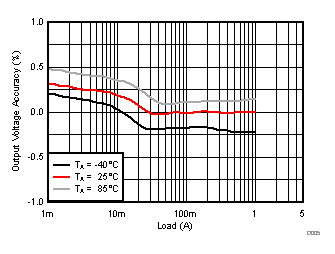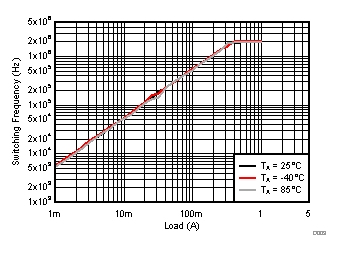VOUT = 1.8V
Figure 22. Switching Frequency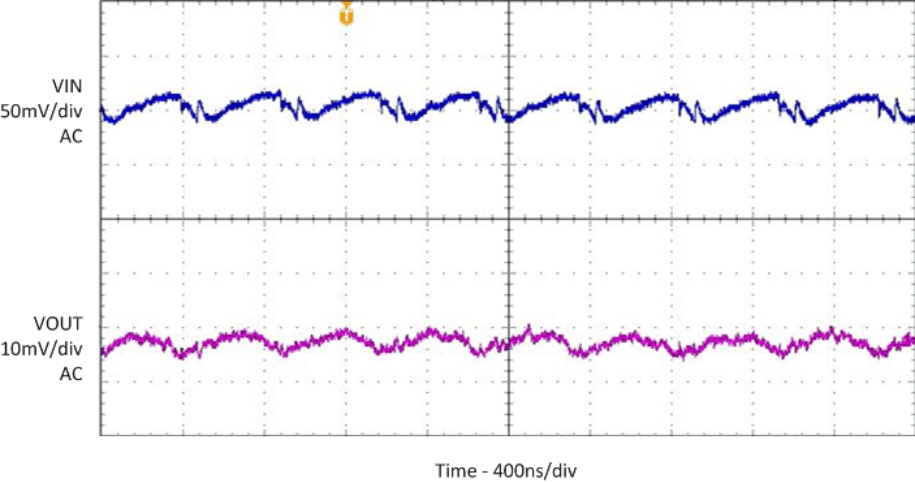IOUT = 1A
Figure 24. Input and Output Ripple in PWM Mode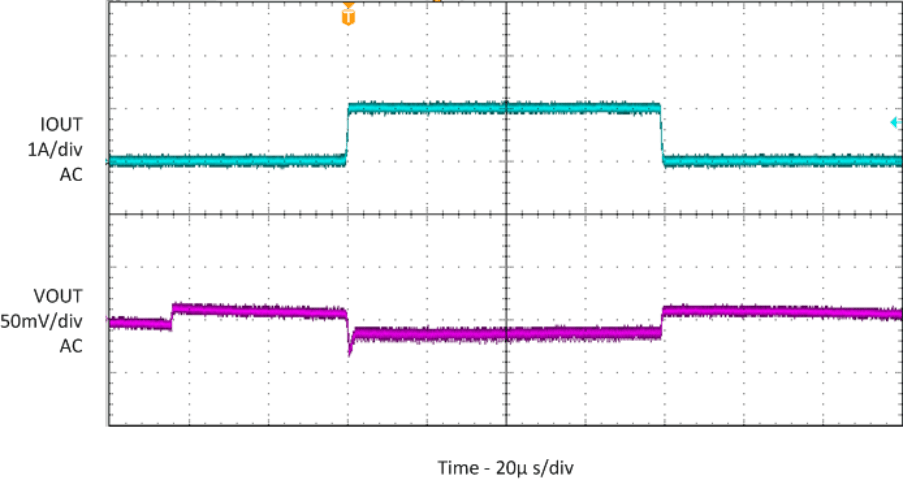IOUT = 0A to 1A, 1A/µs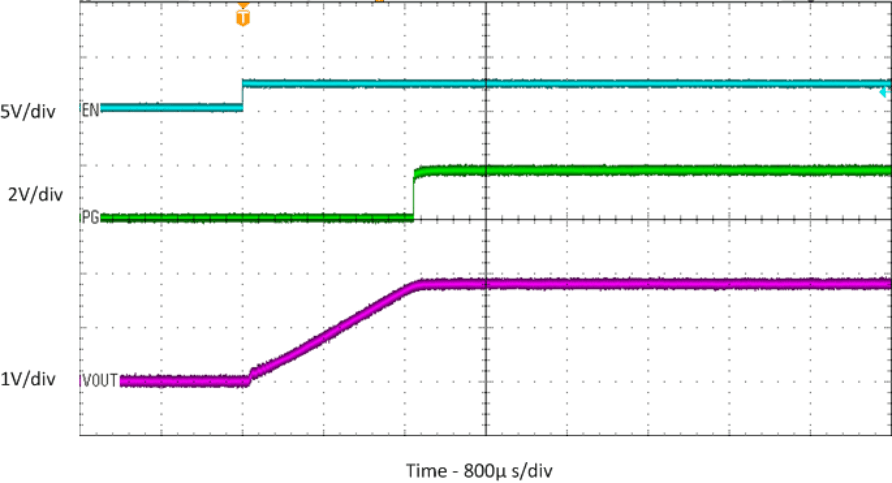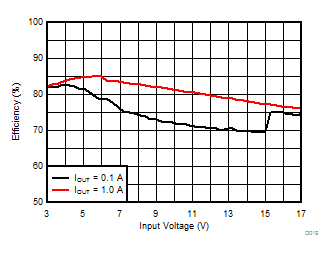Figure 7. Efficiency, VOUT = 1.0 V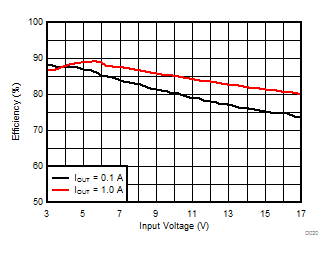Figure 9. Efficiency, VOUT = 1.8 V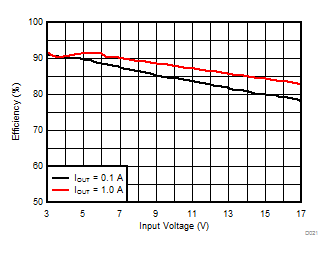Figure 11. Efficiency, VOUT = 2.5 V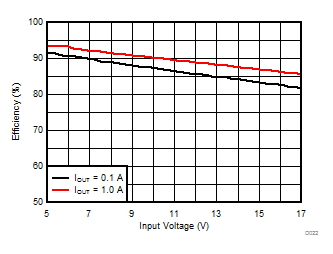Figure 13. Efficiency, VOUT = 3.3 V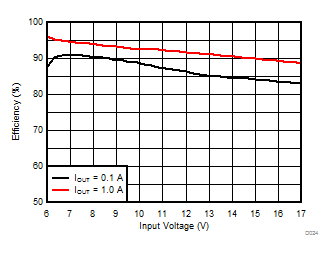Figure 15. Efficiency, VOUT = 5.0 VθJA = 46.1 °C/W
Figure 17. Thermal Derating, VOUT = 1.8 V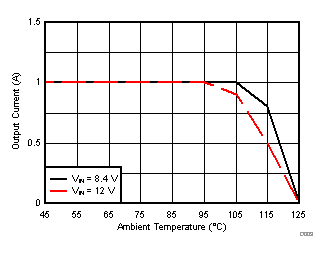θJA = 46.1 °C/W
Figure 19. Thermal Derating, VOUT = 5 V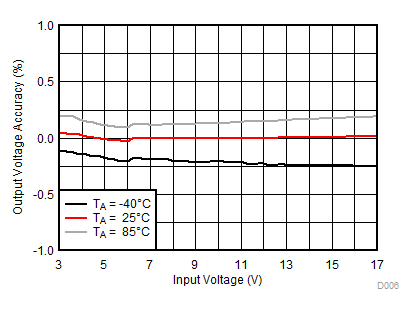IOUT = 1A
Figure 21. Line Regulation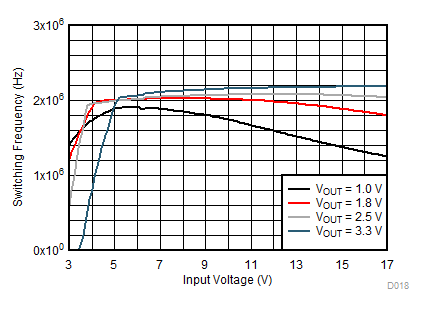IOUT = 1A
Figure 23. Switching Frequency